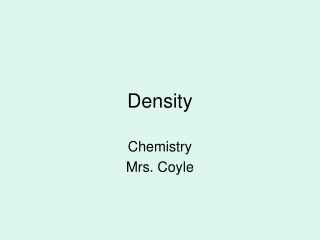DownloadDownload PresentationDensity

# Density

Télécharger la présentation## Density

- - - - - - - - - - - - - - - - - - - - - - - - - - - E N D - - - - - - - - - - - - - - - - - - - - - - - - - - -
##### Presentation Transcript

1. Density Chemistry Mrs. Coyle

2. Density Density= Mass Volume D= m V

3. Mass • Mass: Amount of matter in a substance. • Don’t confuse with weight. • Weight: the force with which the earth pulls on the substance.

4. Question Which weighs more? 50 kilograms of iron Or 50 kilograms of feathers

5. Question Which has a greater density? iron Or feathers

6. Common Units of Density Density= Mass Volume g/mL = g/cm3 kg/m3 1 cm3=1 mL 1 dm3=1L

7. Densities of Common Substances

8. Density as a Function of Temperature For most substances, as temperature increases the volume increases and as a result the density decreases.

9. Density of Water

10. Density of Water At 4oC water has its maximum density of 1g/cm3 Convert to kg/m3: 1000kg/m3

11. Note • Density is an intensive physical property. (Does not depend on amount of matter in the sample)

12. Density Column

13. Galilean Thermometer